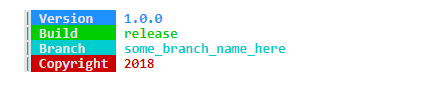# Colors in terminal output

• Does QTextStream provides an out of box solution for colorful output?

I looked a bit and it seems I'll have to make one myself :/

• Colorful Output in Terminal is only possible, if the Terminal supports it. On Linux you have ncurses Library to do that.

• I don't think that's available with QTextStream, however, on Windows, you can achieve this quite easily using GetStdHandle() and SetConsoleTextAttribute() from Windows API .

Example:

``````#ifdef WIN32
//Get std handles
HANDLE hE = GetStdHandle(STD_ERROR_HANDLE);
HANDLE hO = GetStdHandle(STD_OUTPUT_HANDLE);

//Open streams
QTextStream out(stdout);
QTextStream err(stderr);

//Set color
//Standard output --> BLUE
SetConsoleTextAttribute(hO, 0x09);
//Error output --> RED
SetConsoleTextAttribute(hE, 0x0C);
#endif
//Print some messages
out << qPrintable("my message") << endl;
err << qPrintable("error !") << endl;

#ifdef WIN32
//Restore default colors (background: BLACK, foreground: WHITE)
SetConsoleTextAttribute(hO, 0x0F);
SetConsoleTextAttribute(hE, 0x0F);
#endif
``````

Note that the first hexadecimal digit of the value is the background, and the second digit is the foreground, so you can make any combination you want.

Here is the color codes in hexadecimal:
0 = black
1 = navy
2 = green
3 = teal
4 = maroon
5 = purple
6 = olive
7 = silver
8 = gray
9 = blue
A = lime
B = aqua
C = red
D = fuchsia
E = yellow
F = white• If you start your program from inside a terminal which can handle ANSI-Color, you can just print out your text by following the https://en.m.wikipedia.org/wiki/ANSI_escape_code

f.e.

``````#define STD_OUT QTextStream(stdout)

...

QString qsVersion = "1.0.0";
QString  qsBuildInfo = "debug";
#ifdef _DEBUG
qsBuildInfo = "debug";
#else
qsBuildInfo = "release";
#endif
QString qsBranchName = "some_branch_name_here";

STD_OUT << endl << flush;
STD_OUT << QString("  \033[47m|\033[0m\033[1;37;44m Version   \033[0m \033[1;34m%1\033[0m").arg(qsVersion) << endl;
STD_OUT << QString("  \033[47m|\033[0m\033[1;37;42m Build     \033[0m \033[1;32m%1\033[0m").arg(qsBuildInfo) << endl;
STD_OUT << QString("  \033[47m|\033[0m\033[1;37;46m Branch    \033[0m \033[1;36m%1\033[0m").arg(qsBranchName) << endl;
STD_OUT << QString("  \033[47m|\033[0m\033[1;37;41m Copyright \033[0m \033[1;31m%1\033[0m").arg(QDate::currentDate().year()) << endl;
STD_OUT << endl << flush;
``````

resulting in following output: